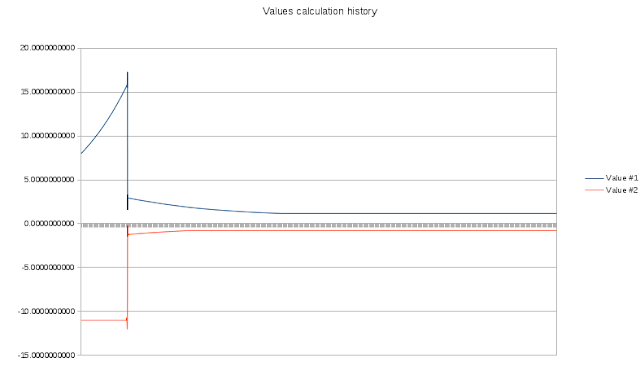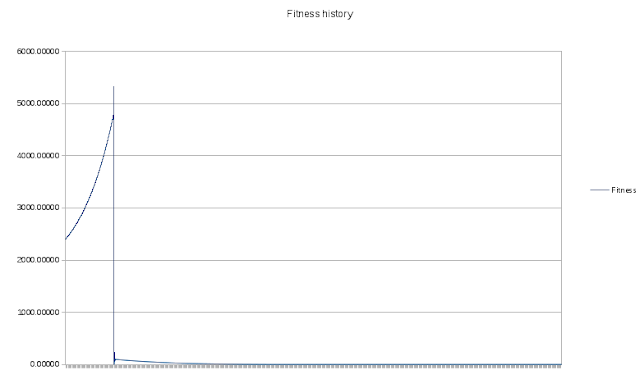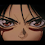## Thursday, January 10, 2013

### Genetic Algorithms. Lame Example - Solving Quadratic Equation

There are numerous resources on the Internet, that provide description of the theory of Genetic Algorithms and theoretical explanation thereof. I, however, have found a bit more then none giving a real example (I may have not searched that good, though). Therefore, I decided to try and implement the theory into a live example. While there are thousands of areas where GA may be applied, I decided to choose trivial quadratic equation solution process for the sake of simplicity of the implementation. The equation is y = 13x^2 - 5x - 12. It has two roots (points where its graph crosses the X axis) at x = -0.7875184 and at x = 1.17213378. Although, this particular GA implementation has no application in real life (you can solve that equation on paper in several seconds), it demonstrates GA at its finest.

Data Encoding
Classic implementation of GA implies encoding of data as bit strings (chromosomes where each gene is represented by one or more bits), however, I decided to change it a bit (which is not prohibited) and use real numbers instead of the bit strings. Each chromosome includes two genes, two possible solutions, one for each root of the equation.

typedef struct _CHROMO_
{
double   value1, value2;
double   fitness;
}chromo_t;

The third member of the chromosome is fitness and is used only to store the fitness of the solution represented by this chromosome. The genes (values) are initiated to random values when the initial population is created:

#define POPULATION 2000

chromot_t population[POPULATION];

for(int i = 0; i < POPULATION; i++)
{
population[i].value1 = (double)(rand() % 10) + 5.0;
population[i].value2 = -(double)(rand() % 10) - 5.0;
}

Fitness Function
Fitness function is used to estimate the suitability of the current solution. In this particular case, the fitness function is the the sum of values produced by the equation when fed the values from the chromosome. This means that the lower the value returned by the fitness function the closed we get to the proper solution.

double fitness(chromo_t* ch)
{
return fabs(13.0 * pow(ch->value1, 2.0) - 5.0 * ch->value1 - 12.0) + fabs(13.0 * pow(ch->value2, 2.0) - 5.0 * ch->value2 - 12.0);
}

While you iterate through the population calculating fitness for each phenotype, you should save the indices of two solutions (the amount may actually vary from case to case) as those would be used to produce the next generation.

Crossover
Crossover operator is the crucial part of any GA implementation. It is a function that takes two (or more) best phenotypes and uses them to produce a new generation. Suggestion is to crossover that pair to produce one (or more - depends on the implementation) child and then crossover the best phenotype with the rest of the population, thus, entirely replacing the old generation with the new one. In this case, the crossover function is rather trivial:

void cross(chromo_t* chMom, chromo_t* chDad, chromo_t* chChild)
{
static int  mutation = 0; // Which operation to use for crossover
switch(mutation)
{
case 0:
chChild->value1 = chMom->value1 + chDad->value1 * MUTATION_RATE;
chChild->value2 = chMom->value2 + chDad->value2 * MUTATION_RATE;
break;

case 1:
chChild->value1 = chMom->value1 + chDad->value1 * MUTATION_RATE;
chChild->value2 = chMom->value2 - chDad->value2 * MUTATION_RATE;
break;

case 3:
chChild->value1 = chMom->value1 - chDad->value1 * MUTATION_RATE;
chChild->value2 = chMom->value2 + chDad->value2 * MUTATION_RATE;
break;

case 4:
chChild->value1 = chMom->value1 - chDad->value1 * MUTATION_RATE;
chChild->value2 = chMom->value2 - chDad->value2 * MUTATION_RATE;
break;

case 5:
chChild->value1 = chDad->value1 - chMom->value1 * MUTATION_RATE;
chChild->value2 = chDad->value2 - chMom->value2 * MUTATION_RATE;
break;

case 6:
chChild->value1 = chDad->value1 + chMom->value1 * MUTATION_RATE;
chChild->value2 = chDad->value2 - chMom->value2 * MUTATION_RATE;
break;

case 7:
chChild->value1 = chDad->value1 - chMom->value1 * MUTATION_RATE;
chChild->value2 = chDad->value2 + chMom->value2 * MUTATION_RATE;
break;
}
mutation++;
if(mutation > 7)
mutation = 0;
}

where the MUTATION_RATE is the speed at which solutions evolve (in this example set to 0.0001). The higher the MUTATION_RATE, the faster you may get to the proper solution, however, it may be less accurate. The best would be to reduce it while approaching the solution.

As you may have noticed, crossover and mutation are combined in a single function, while most of the time you would want to separate them and use mutation only in case of stagnation (inability of the phenotypes to evolve into a proper solution).

Stop Condition
Not much can be said here.  You should stop the execution once you reach desired precision or once you realize that there is no solution as it is totally possible that certain quadratic equation has no roots, instead, it has its minimum or maximum. It is important to mention, that the precision and speed of certain GA implementation depends on the mutation rate and amount of phenotypes in population. In fact, you may implement another GA in order to find optimal values for the current one :).

Testing the Implemented Algorithm
This specific implementation approaches the roots of the aforementioned quadratic equation in a bit less then 23 seconds. Generated solutions are precise enough for most needs.

Let us take a look at the evolution of the solutions generated by the described implementation of GA.This graph shows how solutions change over the execution time.

The fitness curve looks quite similar:Fitness curve
As you may see, GA is capable of finding the right direction in a very fast manner.

Conclusion
While this specific implementation is trivial and, to be honest, quite lame, I hope it shows that GAs are a very powerful tool. It is sage to say, that solving a quadratic equation is one of the simplest tasks where GA may be successfully applied. Genetic Algorithms of different kinds may be used for selection of Artificial Neural Network topology, different algorithm optimizations, etc. The success of certain GA only depends on the correctness of the chromosome and crossover implementations. Let me reiterate - it is always possible to implement another GA in order to get proper implementation of the current one.

Hope this post was helpful. See you at the next.

1.In Linux --> segmentation fault!
My name's MikyGonzalez, regards :)

1.In deed, it fails with segmentation fault on 32 bit systems while it works great on 64 bit (it was written on 64 bit Linux). Add -Os option to the gcc command line to solve it.

2.In fact, I'm on 64-bit linux. Just in case I added -Os but still gives me the error: Segmentation fault (core dumped)

1.I have no idea what the problem is then. It works perfectly for me on my system. Get some more info. Run it with gdb and tell me where the problem occurs

2.I do not manage fine with gdb (debugger'm not used to Linux) but it says:
Program received signal SIGSEGV, Segmentation fault.
0x0000000000400804 in main ()

When I do step by step instruction:
Single stepping Until exit from function main,
Which has no line number information.

3.Compile it with -ggdb, then gdb ./ga2, then run, then bt when it stalls

3.Program received signal SIGSEGV, Segmentation fault.
0x0000000000400804 in main () at main.c:51
51 printf("%d, Best: %4d = %0.40lf, %0.40lf fitness = %0.50lf\n", i, indexBest, population[indexBest].value1, population[indexBest].value2, population[indexBest].fitness);

4.Хороший пост!
Но в качестве наглядных примеров оно как-то больше для machine learning подходит, или whitebox-фаззинга с полиморфными вирусами на худой конец.

5.Hello , I have the source of a rootkit , made in C++ you want to see ? , But it is detected by most anti-virus with a little modification you can make it work again : undetectable ...

1.two questions:
1. Why would I want to see that rootkit?

6.It was not bad at all , bro only i wanted to share so that you can, check out a look or serve in a future ..it is detected by the antivirus ..i can give you the blog from the original user who created it programd in C++ with a little modification you can again make undetectable ,you share because you know the C++ language

1.Thanks, but I am not interested in that. Mainly for two reasons:
1. I do not deal with malware and I have no intention to deal with it in the future;
2. I do not like C++

Oh, and one more point - I do not think C++ is appropriate for writing malware.

7.This comment has been removed by a blog administrator.

8.Hey, This one thing I cannot understand.

double fitness(chromo_t* ch)
{
return fabs(13.0 * pow(ch->value1, 2.0) - 5.0 * ch->value1 - 12.0) + fabs(13.0 * pow(ch->value2, 2.0) - 5.0 * ch->value2 - 12.0);
}

value2 is basically negative value1.
So why have you substituted value1 and value2 in the equation and then added them?
As in why are you computing their sum?

1.It is as simple as:
f = fabs(13x1^2 - 5x1 - 12) + fabs(13x2^2 - 5x2 - 12)
where x1 and x2 are the value1 and value2 of the chromosome.

This means that in order to calculate the fitness of a given chromosome, we simply put its value1 and value2 into the equation.

2.Hey and thanks for the quick reply. I understand now, by keeping value2 negative you ensure that you find the negative root.
thanks again

3.To be more precise, I ensure that they are not the same (unless that fits the fitness function ;) )

9.I mean, how do you know that both value1 and value2 will not turn out to be the same.

10.i cant execute this code, any equation tested is displaying the same result in all generations. For example, in the generate 2000 the two values ​​are 12 and -7 to any equation tested. Can you help me??

1.Do you have any example of an equation you tested that gave you such result?

2.y = 13x ^ 2 – 5x – 12 and
y = x ^2 -X - 2

3.I have just tested it with the same code:
for y=x^2-x-2 the values are approximately 2 and -1 and the execution stops at generation 26830
for 13x^2-5x-12 they are 1.17 and -0.79, generation 69667

I would suggest checking the modifications you're making to the fitness function.

4.can you give all the code please??

5.There's a link at the top of the page. Right under the title of the article.

6.Thanks man!!

11.That link of source code at the top of the page..right under title is not working..

1.It does. But, anyway, here it is http://sites.google.com/site/lyashko/ga.tar.gz

12.Hi, may i know why did you set the value1 = (double)(rand() % 10) + 5.0; and -(double)(rand() % 10) - 5.0;
I mean why you use double and mudolous to 10 the plus or minus with 5?
tq

1.The answer for +/- is simple - there is a bug in the code and this is a quick solution.
Why double and mod 10 - this is simple as well. We need random values that fit specific range and those values must be of type double. As you know - rand() returns an integer value.

2.Owh I understand now, thank you very much for the reply